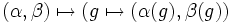# Direct product is cancellative for finite groups

## Statement

Suppose$G, H, K$ are finite groups, such that:$G \times H \cong G \times K$

where$\times$ denotes the external direct product. (Note that the isomorphism need not

Then, we have:$H \cong K$.

## Related facts

### Related facts for other algebraic structures

The statement is true for finite algebras in any variety of algebras:

### Stronger facts for groups

• Krull-Remak-Schmidt theorem: This states something stronger -- there is an analogue of unique factorization for finite groups into directly indecomposable groups. In fact, the result holds for a somewhat more general class of groups: groups that satisfy both the ascending and descending chain conditions on subgroups. This has two important corollaries:
• Corollary of Krull-Remak-Schmidt theorem for cancellation of powers: This states that if$G^m \cong H^m$, and$G$ satisfies both the ascending and descending chain conditions on normal subgroups, then$G \cong H$.
• Corollary of Krull-Remak-Schmidt theorem for cancellation of direct factors: This states the cancellation result for the larger collection of groups, namely those satisfying the ascending and descending chain conditions on normal subgroups.

### Facts for other kinds of products

• Retract not implies normal complements are isomorphic: This basically shows that the analogue of the direct product, namely, the semidirect product, is not right-cancellative for finite groups (it actually shows something stronger).
• Semidirect product is not left-cancellative for finite groups: If$G,H,K$ are finite groups such that$G \rtimes H \cong G \rtimes K$, that does not imply that$H$ is isomorphic to$K$.
• Every group of given order is a permutable complement for symmetric groups: Any group of order$n$ is a permutable complement to$\operatorname{Sym}(n-1)$ inside$\operatorname{Sym}(n)$. Thus, if two subgroups are permutable complements to the same subgroup in the whole group, the only conclusion we can draw is that they gave the same order.

## Facts used

1. Homomorphism set to direct product is Cartesian product of homomorphism sets: If$A,B,C$ are groups, then there is a natural bijection:
•$\operatorname{Hom}(A,B) \times \operatorname{Hom}(A,C) \leftrightarrow \operatorname{Hom}(A,B \times C)$.
• The bijection is defined as:$(\alpha,\beta) \mapsto (g \mapsto (\alpha(g),\beta(g))$.
2. Homomorphism set is disjoint union of injective homomorphism sets: For groups$A$ and$B$, let$\operatorname{Hom}(A,B)$ denotes the set of homomorphisms from$A$ to$B$, and$\operatorname{IHom}(A,B)$ denote the set of injective homomorphisms from$A$ to$B$. Then we have:$\operatorname{Hom}(A,B) = \bigsqcup_{N \triangleleft A} \operatorname{IHom}(A/N, B)$.

## Proof

Given: Finite groups$G, H, K$ such that$G \times H \cong G \times K$.

To prove:$H \cong K$.

Proof: Let$L$ be an arbitrary finite group.

Step no. Assertion/construction Facts used Given data used Previous steps used Explanation
1$|\operatorname{Hom}(L,G \times H)| = |\operatorname{Hom}(L,G)||\operatorname{Hom}(L,H)|$ as an equality of finite numbers. Fact (1)$G,H$ are finite [SHOW MORE]
2$|\operatorname{Hom}(L,G \times K)| = |\operatorname{Hom}(L,G)||\operatorname{Hom}(L,K)|$ as an equality of finite numbers. Fact (1)$G,K$ are finite [SHOW MORE]
3$|\operatorname{Hom}(L,G \times H)| = |\operatorname{Hom}(L,G \times K)|$ as an equality of finite numbers.$G \times H \cong G \times K$ The number of homomorphisms to a group depends only on its isomorphism type.
4$|\operatorname{Hom}(L,H)| = |\operatorname{Hom}(L,K)|$ as an equality of finite numbers. Steps (1),(2),(3) [SHOW MORE]
5 For any finite group$L$, the number of injective homomorphisms from$L$ to$H$ equals the number of injective homomorphisms from$L$ to$K$. We show this by induction on the order of$L$. In other words,$|\operatorname{IHom}(L,H)| = |\operatorname{IHom}(L,K)|$ Fact (2) Step (4) [SHOW MORE]
6$H$ is isomorphic to a subgroup of$K$ and$K$ is isomorphic to a subgroup of$H$$H,K$ are finite Step (5) [SHOW MORE]
7$H$ is isomorphic to$K$$H,K$ are finite Step (6) [SHOW MORE]